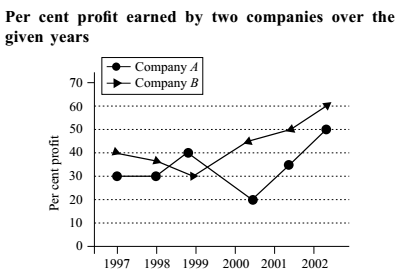## Line chart

#### Data Interpretation

Direction: Study the following graph to answer the given questions.1. If the income of the two companies in 1998 were equal, what was the ratio of their expenditures?

1.  Expenditure of Company A in 1998 ( E1 ) = Income of Company A in 1998 x 100 100 + % profit

 Expenditure of Company B in 1998 ( E2 ) = Income of Company B in 1998 x 100 100 + % profit

Ratio of their expenditures = E1 : E2

##### Correct Option: B

Let the income of the two companies in 1998 be P.

 Expenditure of Company A in 1998 ( E1 ) = P × 100 100 + 35

 Expenditure of Company B in 1998 ( E2 ) = P × 100 100 + 30

 Ratio of their expenditures = E1 : E2 = 100 x 130 = 26 : 27 . 135 100

1. If the income of Company A in 2002 was \$ 600 billion, what was its expenditure?

1. Given in question :- Income of Company A in 2002 = \$ 600 billion
As per given line graph Profit = 60%

 Expenditure of Company A in 2002 = Income of Company A in 2002 x 100 100 + % profit

##### Correct Option: C

Given in question :- Income of Company A in 2002 = \$ 600 billion
As per given line graph Profit = 60%
Use the below formula to calculate the Expenditure.

 Expenditure of Company A in 2002 = Income of Company A in 2002 x 100 100 + % profit

 Expenditure of Company A in 2002 = 600 x 100 = \$ 375 billion 160

1. If the income of Company B and A in 1998 was same, what was its profit in 1999?

1. We can not find out the amount of profit in 1998, we do not know the income and expenditure of A and B.

##### Correct Option: D

We can not find out the amount of profit in 1998, we do not know the income and expenditure of A and B, therefore option (d) is the correct choice.

1. What is the percent increase in profit for company B from year 2000 to 2001?

1.  Required % Increase in Profit = Total increase in profit x 100% Initial profit

 Required % Increase in Profit = Profit in 2001 - Profit in 2000 x 100% Profit in 2000

##### Correct Option: A

 Required % Increase in Profit = Total increase in profit x 100% Initial profit

 Required % Increase in Profit = Profit in 2001 - Profit in 2000 x 100% Profit in 2000

 % Increase in Profit = 35 - 20 x 100 = 75 % 20

1. If the expenditure of Company B in 2000 was \$ 200 billion, what was its income?

1. As per given line graph,
Percentage Profit in 2000 by Company B = 20 %
As per given question,
Expenditure of Company B in 2000 = 200 billion \$
Use the below formula to calculate the Income of company in 2000

 Income of Company B in 2000 = Expenditure of Company B in 2000 x 100 + % Profit 100

##### Correct Option: A

As per given line graph,
Percentage Profit in 2000 by Company B = 20 %
As per given question,
Expenditure of Company B in 2000 = 200 billion \$
Use the below formula to calculate the Income of company in 2000

 Income of Company B in 2000 = Expenditure of Company B in 2000 x 100 + % Profit 100

 Income of Company B in 2000 = 200 x 120 = \$ 240 billion 100